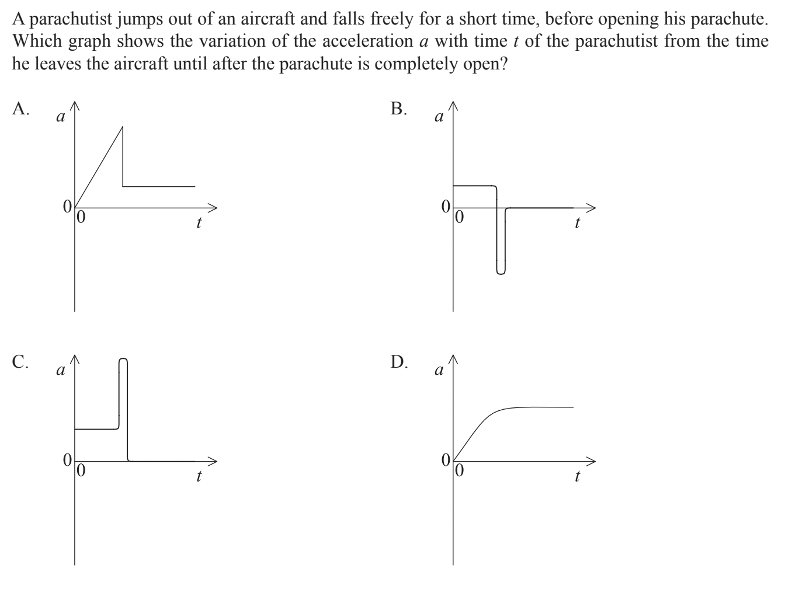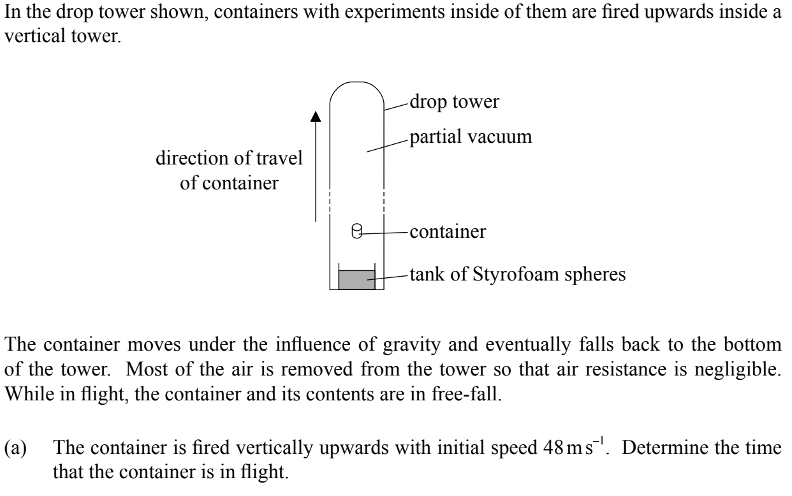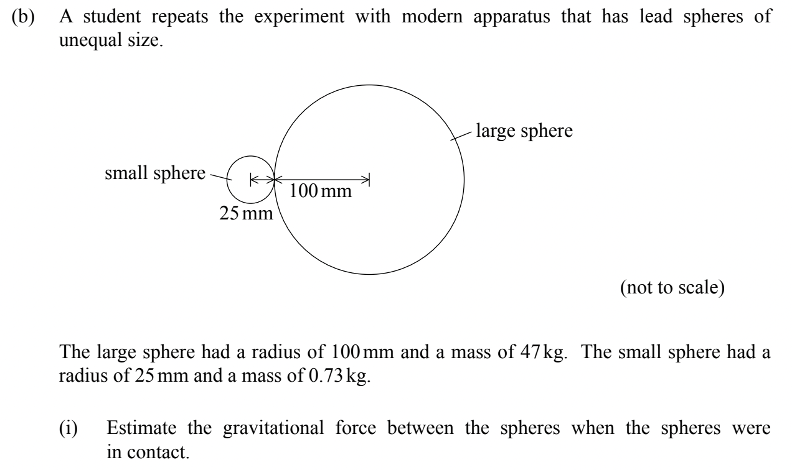### What are your chances of acceptance?Duke UniversityUCLA
Unweighted GPA: 3.7
1.0
4.0
SAT: 720 math
200
800
| 800 verbal
200
800

#### Extracurriculars

Low accuracy (4 of 18 factors)Varun Srinivasan
6 IB Guides

# IB Physics HL Exam Practice Questions

## What’s Covered:

The IB program is considered as rigorous as it is respected. This especially applies to its STEM courses, and Physics HL is no exception. This course is designed for students to study freshman to sophomore year university level physics in high school. The coursework can be difficult but it is one of the best ways to prepare a student for further education in STEM.

This guide is about the examinations involved with IB Physics HL, and how to succeed in them.

## Overview of the IB Physics HL Exam

The IB Physics HL exam is a set of 3 different kinds of papers, all structured and formatted differently in an attempt to test students on different aspects of coursework for the class. The formatting is as such:

• Paper 1 (MCQ) – 40 Marks / 60 minutes long / No calculator permitted
• Paper 2 – 90 Marks / 135 minutes long / Calculator permitted
• Paper 3 – 45 Marks / 75 minutes long / Calculator permitted

These three papers in total will cover most, if not all of the coursework that you’ll go over during your two-year course of Physics HL. You’ll notice almost right away when you start practicing questions from papers that the style of the questions varies from paper to paper. This means different approaches and methods are required to approach different kinds of questions.

## IB Physics HL Exam Questions

The most obvious and efficient way to prepare for any kind of exam, especially a physics exam, is to just practice older examinations and papers. This is a great way to familiarize yourself with the style and formatting of IB exams. This way, you might also be less nervous for the actual exam because you have a rough idea of what to expect.

### Paper 1 – Question 1We can eliminate C because the parachutist can’t have the acceleration reach such a peak because opening the parachute must actually decelerate the parachutist.

A and D imply the parachutist is initially gradually increasing in acceleration, but this is not possible. During free fall on Earth, due to the gravitational constant of acceleration being $$9.8\ ms^{-2}$$, any object in free fall has that exact value for acceleration. Therefore, the value of acceleration can’t gradually rise from 0; it simply begins at 9.8 when in free fall.

B is correct because it indicates the constant acceleration during free fall, as well as a sign of deceleration when the parachute is opened, after which the parachutist reaches an acceleration of 0, which is maintained as the parachutist floats down with constant speed due to air resistance of the parachute.

### Paper 1 – Question 2It could be easier to visualize the point at which tension = 0, where the spring becomes slack. At this point the gain in gravitational potential energy of the mass is the same as the work done on the lifted mass by the student, in addition to the potential energy lost by the string due to loss of tension.

For paper 1 a lot of questions are actually shared between SL and HL. Therefore, it could actually be beneficial for you to also study the SL variants of Physics paper 1. This shouldn’t be a common practice for many other subjects, though, so please make sure to double check the content of exams before trying a SL paper.

Moving on, let’s try some paper 2 questions.

### Paper 2 – Question 1

You might notice the following question is the same as the one we discussed for the IB Physics SL exam. That’s totally common as IB likes to recycle their questions between HL and SL. Just keep in mind that IB holds HL students to a higher standard when grading papers, and this reflects in the mark schemes where they want thorough answers.Because the container is fired upward with its initial speed of 48, it also comes back down with the same speed in free fall. This is because in free fall an object is not increasing in acceleration, therefore maintaining a stable speed due to lack of air resistance (no air resistance can occur in a vacuum).

Therefore we can use the following kinematic equation to figure out time:

$$Final\ Velocity=Initial\ Velocity-9.81t$$

9.81 is the gravitational constant, a value of acceleration due to gravity.

Our initial speed is 48, and our final speed is -48 due to the container returning in the opposite direction in which it came from.

$$-48=48-9.81t$$

You can use a calculator for this paper, and all the kinematic equations you’ll need are found in the data booklet provided during the exam.

$$t=9.8$$

### Paper 2 – Question 2The traveling sound wave is reflected at the point it meets the barrier, and then begins to travel in the opposite direction. At this point the waves of new sound emitted from the speaker and the reflected ones undergo superposition, which means they are overlapping with each other. This causes two forms of interference to take place:

• Destructive interference, which forms the minima of wave intensity
• Constructive interference, which forms the maxima of wave intensity### Paper 2 – Question 3Newton’s law of gravitation is proportional to the product of the masses and is also inversely proportional to the square of the distance between the centers of two masses.$$force=\frac{G\cdot Mass_{1}\cdot Mass_{2}}{radius^2}$$, where $$G$$ is the gravitational constant (the value of $$G$$ is provided in the data booklet) and the radius is the distance between the centers of the two objects.

Given what we are provided with, we know:

$$force=\frac{6.67\cdot10^{-11}\cdot47\cdot0.73}{(1.25\cdot10^{-1})^2}=0.15\mu\ N$$

As you can tell, paper 2 questions are significantly longer and harder than paper 1, even with the use of a calculator. Considering that this paper will make a bulk of your points and grade for the class, try your best to practice for this and excel!

Let’s take a look at a paper 3 question. Please note that this guide will not cover option specific questions, just general ones!

### Paper 3 – Question 1The estimated percentage uncertainty is solved with:

$$2\cdot time\ uncertainty\ percentage+height\ uncertainty\ percentage$$

This equates to $$2\cdot 0.3+0.6=1%$$

The following suggestions could have obtained a more reliable value for $$g$$:

• Use more than one height
• Use a smaller ball to reduce air resistance/drag
• Repeat the experiment and collect an average for more accurate results

Just like paper 1, a lot of questions for paper 3 in Physics SL are the same, if not similar to the SL variant. So it’s totally fine to refer to SL papers for extra practice on similar problems!

## Calculator Suggestions for IB Physics HL

There are a bunch of options for calculators when it comes to IB Physics, but the following are trusted brands and great for making the time-intensive calculations for paper 2 and 3 easier. Of course, any calculator will suffice as long as it’s capable of graphing, so if you don’t like any on this list, feel free to try another one!

• Ti84 plus (all models)
• Ti Nspire (non-CAS models) with the 84 faceplate
• Ti83 plus
• Casio CFX 9850 Plus
• Casio FX 9950 Plus

## Final Tips

All IB exams are hard, and IB Physics HL is a great example of this. IB Physics HL can be intimidating, but it gets less and less scary with more practice! Here are some extra tips to make exam day a bit more manageable.

### Spend Extra Time in the Lab

Paper 3 is a lab-knowledge based paper. A lot of the questions are about scientific techniques and measures taken in labs to improve better data collection and overall lab-procedure. This knowledge isn’t as easily gained through studying a book, something you can get away with for papers 1 and 2. Instead, spend extra time in your school lab and observe the practical elements of the concepts you learn. You’ll make better connections between the coursework and its uses, leading to a mindset to perform better in paper 3.

### Practice SL Questions

Physics HL papers 1 and 3 share lots of questions with their SL variants. This means you can get even more practice by studying SL papers on top of your HL practice. SL papers are also slightly easier so they’re perfect for brushing up on basics or improving your foundation for the course.

## How Does the IB Physics HL Exam Affect My College Chances?

It doesn’t! Your actual grades in IB mean little in admissions, but showing the determination to take difficult classes and sticking with them shows a lot more. This trait is appealing to admissions officers.

If the admissions process seems a little confusing, or you need a better idea on your chances of admission for further education, check out CollegeVine’s free admissions calculator, a tool that takes GPA, extracurriculars, standardized test scores, and more to calculate your unique probability of getting into a school!Short Bio
Varun is a recent graduate from Arizona State University, Tempe, with a degree in Computer Science. He aims to share his knowledge of computer science, the IB Diploma Program, and all things college-related with high school students. In his free time, he can be found performing DJ sets or cooking!